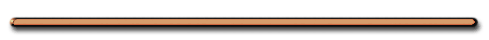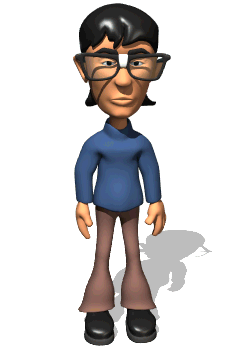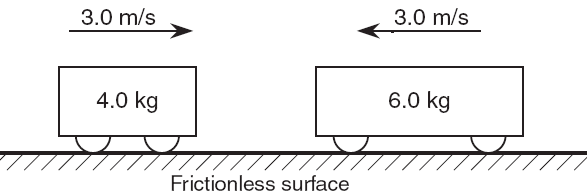Try This at Home

Review - 3 Laws of Motion

II. Conservation of Momentum

[ FLASH ][ HTML5 ]In a Closed System:

When two objects interact,…….

....the SUM of their momenta BEFORE the interaction ...

...equals the SUM of their momenta AFTER the interaction.

 Total Momentum Before Interaction = Total Momentum After Interaction

m1v1 + m2v2 = m1v1′ + m2v2

Totally Elastic Collision?What is the momentum of the big fish after it  swallows the little fish?

[ Flash ]

-4 kgm/s

Total momentum before =
Total momentum after

A) Collision Problems

 Ex 1) A 3.0 kg object traveling 6.0 m/s E has a perfectly elastic collision with a 4.0 kg object traveling 8.0 m/s W.  After the collision, the 3.0 kg object will travel 10. m/s west.  a) Total momentum before the collision?

Ex 1) A 3.0 kg object traveling 6.0 m/s east has a perfectly elastic collision with a 4.0 kg object traveling 8.0 m/s west. After the collision, the 3.0 kg object will travel 10. m/s west.

a) What was the total momentum before the collision?

[ Flash ]

 Before After m1 = 3.0 kg v1 = 6.0 m/s(East) m2 = 4.0 kg v2 = - 8.0 m/s  (West)

Total momentum before:

= m1v1 + m2v2

= 3.0 kg(6.0 m/s)+4 kg(-8 m/s)

= 18. kg(m/s) + -32. kgm/s

= - 14. kg(m/s)

OR

14. kg(m/s) West

b) What is the total momentum of these objects after this collision?

= -14. kg(m/s)

OR 14. kg(m/s) West

Total Momentum Before Interaction = Total Momentum After Interaction

Ex 1) ..... After the collision, the 3.0 kg object will travel 10. m/s west

c) What velocity will the 4.0 kg object have after the collision?

m1 = 3.0 kg

v1′ = -10. m/s

m2 = 4.0 kg

v2′ = ?

Total Momenta Before Interaction = Total Momenta After Interaction

 Before After

-14. kg(m/s) = m1v1′ + m2v2

-14. kg(m/s) =

3.0 kg(-10. m/s)+4.0 kg(v2′)

-14. kg(m/s) =

(-30. kgm/s)+4.0 kg(v2′)

16. kg(m/s) = 4.0 kg(v2′)

v2′ = 4.0 m/s

OR

v2′ = 4.0 m/s EastWhat is the momentum of the attached carts?

 p = 15 kgm/s p = -30 kgm/s

[ Flash ]

Total momentum before = total momentum after

 Ex 2) A 10. kg Block A moves with a velocity of 2.0 m/s to the right and collides with a 10. kg Block B which is at rest.  After the collision Block A stops moving and Block B moves to the right.  a) Find total momentum after the collision.

Ex 2) A 10. kg Block A moves with a velocity of 2.0 m/s to the right and collides with a 10. kg Block B which is at rest.  After the collision Block A stops moving and Block B moves to the right.

a) Find the total momentum after the collision

 Before After mA = 10. kg mA = 10. kg VA = 2.0 m/s (East) VA′ = 0 m/s mB = 10. kg mB = 10. kg VB = 0 m/s VB′ = ?

Total mom. before = Total mom. after:

= mAvA + mBvB

= 10. kg(2.0m/s)+10. kg(0 m/s)

= 20 kgm/s

Ex 2) A 10. kg Block A moves with a velocity of 2.0 m/s to the right and collides with a 10. kg Block B which is at rest.  After the collision Block A stops moving and Block B moves to the right.

Find the velocity of Block B after the collision.

 Before After mA = 10. kg mA = 10. kg vA = 2.0 m/s (East) vA′ = 0 m/s mB = 10. kg mB = 10. kg VB = 0 m/s vB′ = ?

Total mom. before
= Total mom. after:

20. kgm/s = mAVA + mBVB

20. kgm/s =

10. kg(0m/s)+10. kgV2

VB = 2.0 m/s

 Ex 3) A 10. kg cart moving with a velocity of 10. m/s East collides and attaches itself to a 10. kg cart moving at a velocity of  50. m/s west. a) Find the total momentum before the collision

Ex 3) A 10. kg cart moving with a velocity of 10. m/s East collides and attaches itself to a 10. kg cart moving at a velocity of  50. m/s west.

a) Find the total momentum before the collision

 Before After mA = 10. kg vA = 10. m/s(East) mB = 10. kg vB = - 50. m/s

Total momentum = m1v1 + m2v2

Total momentum =

100. kgm/s + -500. kgm/s

= -4.0 x 102 kgm/s

Impulse

Hi-speed video of a golf ball compressed by driver 00:06 - 3 years ago

Recorded at 10,000 fps with the Photron ultima APX slow motion video camera, the gold ball can be seen to compress as the golf club comes into contact with it at high speed. Recorded with the APX as 10K fps, with a 10 microsecond shutter and 512 (H) x 256 (V) at 10-bit pixel depth.

Ex 3) A 10. kg cart moving with a velocity of 10. m/s East collides and attaches itself to a 10. kg cart moving at a velocity of  50. m/s west.

b) Find the total momentum after the collision

Total mom. before
= Total mom. after:

= -4.0 x 102 kgm/s

Ex 3) A 10. kg cart moving with a velocity of 10. m/s East collides and attaches itself to a 10. kg cart moving at a velocity of  50. m/s west.

c) What is the velocity of the attached carts after the collision?

 m = 20. kg (masses combine) v = ?

Total mom. before =
Total mom. after:

-400. kgm/s  = (20. kg)V

V = -20. m/s

V = 20. m/s W

 Ex 4) What is the magnitude of the total momentum of these carts?Total momentum = m1v1 + m2v2

Total mom. =

(4.0 kg)(3 m/s)+6.0kg(-3.0m/s)

= 12. kgm/s + -18. kgm/s

= -6.0 kgm/s"Left train momentum = 30 kgm/s. What is the momentum  of the right train?"

What's the total momentum before the collision?

After the collision?

[ Flash ]

AP Physics

Does this obey the conservation of momentum?

[ Flash ]

[AP]Recoil Problems